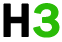C++基础 专题

C++ 数字

## c++ 定义数字

``````#include <iostream>
using namespace std;

int main ()
{
// 数字定义
short  s;
int    i;
long   l;
float  f;
double d;

// 数字赋值
s = 10;
i = 1000;
l = 1000000;
f = 230.47;
d = 30949.374;

// 数字输出
cout << "short  s :" << s << endl;
cout << "int    i :" << i << endl;
cout << "long   l :" << l << endl;
cout << "float  f :" << f << endl;
cout << "double d :" << d << endl;

return 0;
}``````

```short  s :10
int    i :1000
long   l :1000000
float  f :230.47
double d :30949.4
```

## c++ 数学运算

c++ 内置了丰富的数学函数，可对各种数字进行运算。下表列出了 c++ 中一些有用的内置的数学函数。

1 double cos(double);

2 double sin(double);

3 double tan(double);

4 double log(double);

5 double pow(double, double);

6 double hypot(double, double);

7 double sqrt(double);

8 int abs(int);

9 double fabs(double);

10 double floor(double);

``````#include <iostream>
#include <cmath>
using namespace std;

int main ()
{
// 数字定义
short  s = 10;
int    i = -1000;
long   l = 100000;
float  f = 230.47;
double d = 200.374;

// 数学运算
cout << "sin(d) :" << sin(d) << endl;
cout << "abs(i)  :" << abs(i) << endl;
cout << "floor(d) :" << floor(d) << endl;
cout << "sqrt(f) :" << sqrt(f) << endl;
cout << "pow( d, 2) :" << pow(d, 2) << endl;

return 0;
}``````

```sign(d) :-0.634939
abs(i)  :1000
floor(d) :200
sqrt(f) :15.1812
pow( d, 2 ) :40149.7
```

## c++ 随机数

``````#include <iostream>
#include <ctime>
#include <cstdlib>

using namespace std;

int main ()
{
int i,j;

// 设置种子
srand( (unsigned)time( null ) );

/* 生成 10 个随机数 */
for( i = 0; i < 10; i++ )
{
// 生成实际的随机数
j= rand();
cout <<"随机数： " << j << endl;
}

return 0;
} ``````

```随机数： 1748144778

```